Shortcuts

# R2 Score¶

## Module Interface¶

Computes r2 score also known as R2 Score_Coefficient Determination: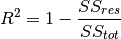where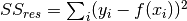is the sum of residual squares, and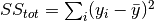is total sum of squares. Can also calculate adjusted r2 score given by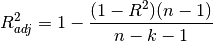where the parameter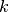(the number of independent regressors) should be provided as the adjusted argument.

As input to forward and update the metric accepts the following input:

• preds (Tensor): Predictions from model in float tensor with shape (N,) or (N, M) (multioutput)

• target (Tensor): Ground truth values in float tensor with shape (N,) or (N, M) (multioutput)

As output of forward and compute the metric returns the following output:

In the case of multioutput, as default the variances will be uniformly averaged over the additional dimensions. Please see argument multioutput for changing this behavior.

Parameters
• num_outputs (int) – Number of outputs in multioutput setting

• adjusted (int) – number of independent regressors for calculating adjusted r2 score.

• multioutput (str) –

Defines aggregation in the case of multiple output scores. Can be one of the following strings:

• 'raw_values' returns full set of scores

• 'uniform_average' scores are uniformly averaged

• 'variance_weighted' scores are weighted by their individual variances

• kwargs (Any) – Additional keyword arguments, see Advanced metric settings for more info.

Raises
• ValueError – If adjusted parameter is not an integer larger or equal to 0.

• ValueError – If multioutput is not one of "raw_values", "uniform_average" or "variance_weighted".

Example

>>> from torchmetrics import R2Score
>>> target = torch.tensor([3, -0.5, 2, 7])
>>> preds = torch.tensor([2.5, 0.0, 2, 8])
>>> r2score = R2Score()
>>> r2score(preds, target)
tensor(0.9486)

>>> target = torch.tensor([[0.5, 1], [-1, 1], [7, -6]])
>>> preds = torch.tensor([[0, 2], [-1, 2], [8, -5]])
>>> r2score = R2Score(num_outputs=2, multioutput='raw_values')
>>> r2score(preds, target)
tensor([0.9654, 0.9082])


Initializes internal Module state, shared by both nn.Module and ScriptModule.

## Functional Interface¶

Computes r2 score also known as R2 Score_Coefficient Determination:whereis the sum of residual squares, andis total sum of squares. Can also calculate adjusted r2 score given bywhere the parameter(the number of independent regressors) should be provided as the adjusted argument.

Parameters
• preds (Tensor) – estimated labels

• target (Tensor) – ground truth labels

• adjusted (int) – number of independent regressors for calculating adjusted r2 score.

• multioutput (str) –

Defines aggregation in the case of multiple output scores. Can be one of the following strings:

• 'raw_values' returns full set of scores

• 'uniform_average' scores are uniformly averaged

• 'variance_weighted' scores are weighted by their individual variances

Raises
• ValueError – If both preds and targets are not 1D or 2D tensors.

• ValueError – If len(preds) is less than 2 since at least 2 sampels are needed to calculate r2 score.

• ValueError – If multioutput is not one of raw_values, uniform_average or variance_weighted.

• ValueError – If adjusted is not an integer greater than 0.

Example

>>> from torchmetrics.functional import r2_score
>>> target = torch.tensor([3, -0.5, 2, 7])
>>> preds = torch.tensor([2.5, 0.0, 2, 8])
>>> r2_score(preds, target)
tensor(0.9486)

>>> target = torch.tensor([[0.5, 1], [-1, 1], [7, -6]])
>>> preds = torch.tensor([[0, 2], [-1, 2], [8, -5]])
>>> r2_score(preds, target, multioutput='raw_values')
tensor([0.9654, 0.9082])

Return type

Tensor

© Copyright Copyright (c) 2020-2023, Lightning-AI et al... Revision 6853c553.

Built with Sphinx using a theme provided by Read the Docs.
Versions
latest
stable
v0.11.2
v0.11.1
v0.11.0
v0.10.3
v0.10.2
v0.10.1
v0.10.0
v0.9.3
v0.9.2
v0.9.1
v0.9.0
v0.8.2
v0.8.1
v0.8.0
v0.7.3
v0.7.2
v0.7.1
v0.7.0
v0.6.2
v0.6.1
v0.6.0
v0.5.1
v0.5.0
v0.4.1
v0.4.0
v0.3.2
v0.3.1
v0.3.0
v0.2.0
v0.1.0